Function Repository Resource:

Divide an image into a list of colored polygons centered around the origin

Contributed by: Erik Mahieu
 ResourceFunction["ImageRadialDivide"][img] divides the image img into polygons centered around the origin.

## Details and Options

An image of width w is divided into w/2 rings of w polygons each, resulting in w2/2 colored polygons.
Both face and edge colors are taken to be the color of the pixel closest to the centroid of the polygon in the image.
The result is a disk-shaped image where pixels outside of the disk region are discarded.
ResourceFunction["ImageRadialDivide"] is very important for transformations of an image that operate inhomogeneously, that is, differently on different parts of the image. This is the case with anamorphic transformations where the deformation of the original is dependent on the locations of each pixel.

## Examples

### Basic Examples (1)

Radially divide an image into a list of colored polygons: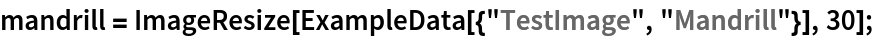In:=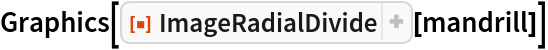Out=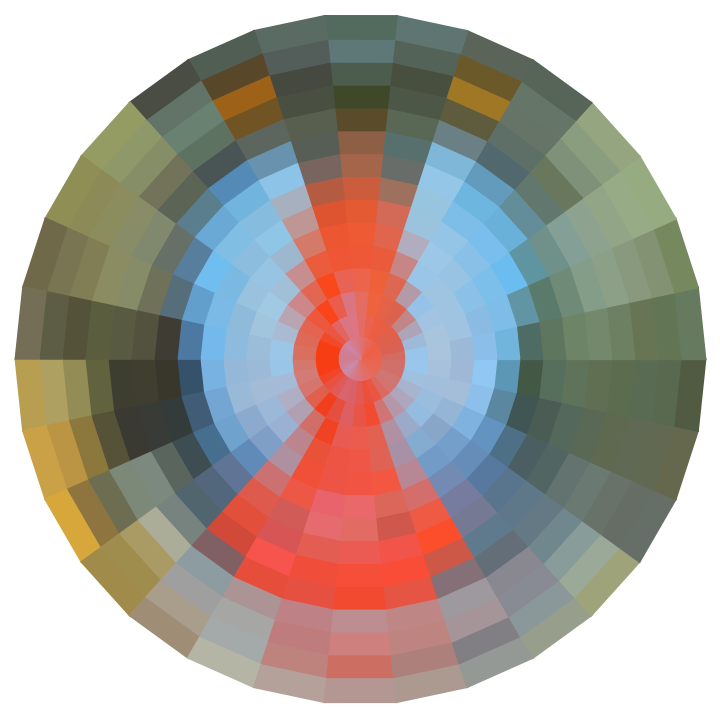### Scope (2)

The output of the function is a list of colored polygons:

 In:=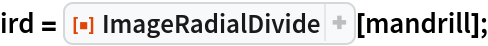In:=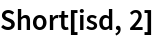Out=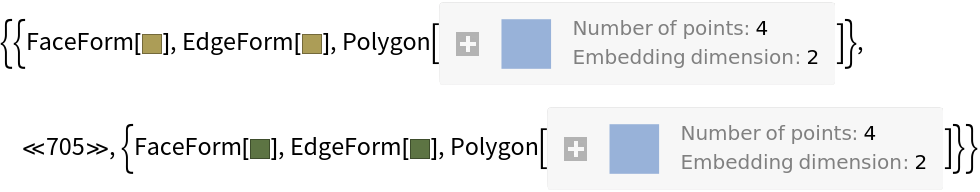In:=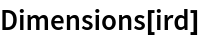Out=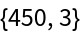The function operates best on circular images such as logos and the like:

 In:=In:=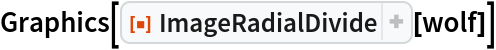Out=### Neat Examples (1)

Here is an example of a conical mirror anamorphic function operating on the vertices of an image that was divided into squares:

 In:=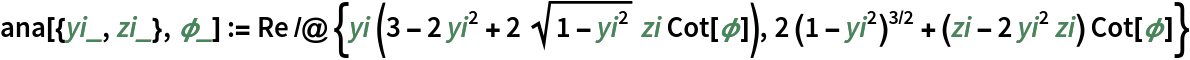In:=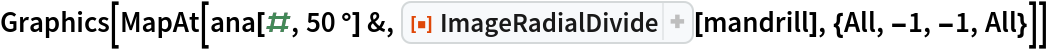Out=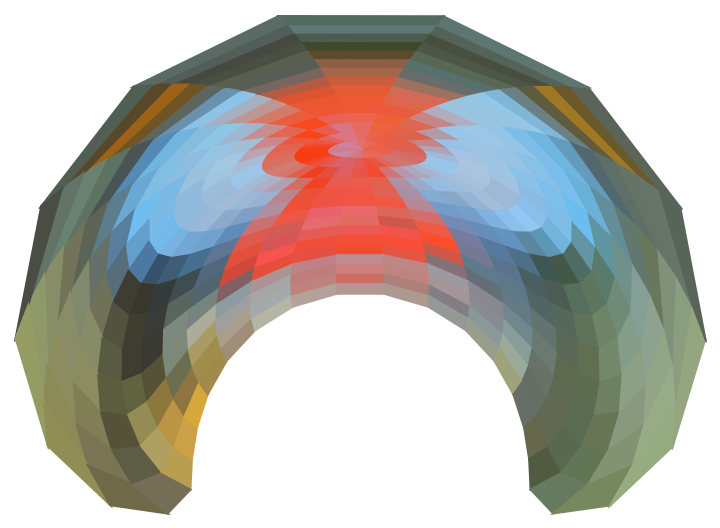Erik Mahieu

## Version History

• 1.0.0 – 09 November 2020